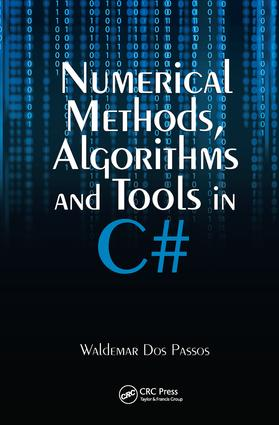Numerical Methods, Algorithms and Tools in C#

1st Edition

CRC Press

598 pages | 3 B/W Illus.

Purchasing Options:\$ = USD
Hardback: 9780849374791
pub: 2009-10-23
SAVE ~\$28.00
\$140.00
\$112.00
x
eBook (VitalSource) : 9780429128547
pub: 2016-04-19
from \$28.98

FREE Standard Shipping!

Description

Comprehensive Coverage of the New, Easy-to-Learn C#

Although C, C++, Java, and Fortran are well-established programming languages, the relatively new C# is much easier to use for solving complex scientific and engineering problems. Numerical Methods, Algorithms and Tools in C# presents a broad collection of practical, ready-to-use mathematical routines employing the exciting, easy-to-learn C# programming language from Microsoft.

The book focuses on standard numerical methods, novel object-oriented techniques, and the latest Microsoft .NET programming environment. It covers complex number functions, data sorting and searching algorithms, bit manipulation, interpolation methods, numerical manipulation of linear algebraic equations, and numerical methods for calculating approximate solutions of non-linear equations. The author discusses alternative ways to obtain computer-generated pseudo-random numbers and real random numbers generated by naturally occurring physical phenomena. He also describes various methods for approximating integrals and special functions, routines for performing statistical analyses of data, and least squares and numerical curve fitting methods for analyzing experimental data, along with numerical methods for solving ordinary and partial differential equations. The final chapter offers optimization methods for the minimization or maximization of functions.

Exploiting the useful features of C#, this book shows how to write efficient, mathematically intense object-oriented computer programs. The vast array of practical examples presented can be easily customized and implemented to solve complex engineering and scientific problems typically found in real-world computer applications.

Reviews

"…the book does present some clear and neat classes and methods that implement the standard algorithms. …The advantage of owning a copy of this book is to have these classical algorithms collected together on your bookshelf in a language that you want to use. …"

—I-Programmer, December 2009

Introduction

C# and the .NET Framework

Installing C# and the .NET Framework

Overview of Object-Oriented Programming (OOP)

Your First C# Program

Overview of the IDE Debugger

Overview of the C# Language

The .NET Framework Math Class Library

Introduction

A .NET Framework Math Class—Fields

A .NET Framework Math Class—Methods

Vectors and Matrices

Introduction

The C# Vector Library

The C# Matrix Library

Complex Numbers

Introduction

Fundamental Concepts

Complex Number Arithmetic

Elementary Functions of a Complex Number

A Complex Number Library in C#

Complex Number Vectors

Complex Number Matrices

Generic vs. Non-Generic Coding

Sorting and Searching Algorithms

Introduction

Sorting Algorithms

Comparison Sorts

Count Sort

Search Algorithms

Bits and Bytes

Introduction

Numeric Systems

Bit Manipulation and Bitwise Operators

Assorted Bits and Bytes

Interpolation

Introduction

Linear Interpolation

Bilinear Interpolation

Polynomial Interpolation

Cubic Spline Interpolation

Linear Equations

Introduction

Gaussian Elimination

Gauss-Jordan Elimination

LU Decomposition

Iteration Methods

Eigenvalues and Jacobi’s Algorithm

Non-Linear Equations

Introduction

Linear Incremental Method

Bisection Method

The Secant Method

False Positioning Method

Fixed Point Iteration

Newton–Raphson Method

Random Numbers

Introduction

The C# Built-In Random Number Generator

Other Random Number Generators

True Random Number Generators

Probability Distribution Functions

Histograms

Discrete Distributions

Continuous Distributions

Shuffling Algorithms

Numerical Differentiation

Introduction

Finite Difference Formulas

Richardson Extrapolation

Derivatives by Polynomial Interpolation

Numerical Integration

Introduction

Newton-Cotes Formulas

Romberg Integration

Monte Carlo Methods

Convolution Integrals

Statistical Functions

Introduction

Some Useful Tools

Basic Statistical Functions

Special Functions

Introduction

Factorials

Combinations and Permutations

Gamma Function

Beta Function

Error Function

Sine and Cosine Integral Functions

Laguerre Polynomials

Hermite Polynomials

Chebyshev Polynomials

Legendre Polynomials

Bessel Functions

Curve Fitting Methods

Introduction

Least Squares Fit

Weighted Least Squares Fit

Linear Regression

The x2 Test for Goodness of Fit

Ordinary Differential Equations

Introduction

Euler Method

Runge–Kutta Methods

Coupled Differential Equations

Partial Differential Equations

Introduction

The Finite Difference Method

Parabolic Partial Differential Equations

Hyperbolic Partial Differential Equations

Elliptic Partial Differential Equations

Optimization Methods

Introduction

Linear Programming

Simulated Annealing Method

Genetic Algorithms

References

Index

Waldemar Dos Passos is a computer programming consultant in the Silicon Valley area of California. After completing his undergraduate education at the University of California, Berkeley, Dr. Dos Passos earned an M.S. in computer science and engineering along with a Ph.D. in physics from the University of Michigan, Ann Arbor. With more than twenty years of computer programming experience, he has published several papers in physics journals.